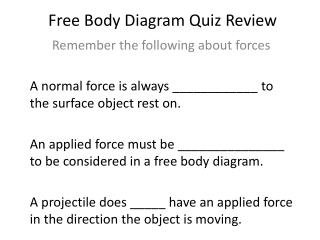Download PresentationFree Body Diagram Quiz Review

# Free Body Diagram Quiz Review - PowerPoint PPT PresentationDownload Presentation## Free Body Diagram Quiz Review

- - - - - - - - - - - - - - - - - - - - - - - - - - - E N D - - - - - - - - - - - - - - - - - - - - - - - - - - -
##### Presentation Transcript

1. Free Body Diagram Quiz Review Remember the following about forces A normal force is always ____________ to the surface object rest on. An applied force must be _______________ to be considered in a free body diagram. A projectile does _____ have an applied force in the direction the object is moving.

2. Free Body Diagram Quiz Review Remember the following about forces When an object is moving along a surface, the _____________________must be added unless otherwise stated. Gravitational force always has an acceleration of _____________. Gravitational force is always ________ down.

3. Free Body Diagram Quiz Review Remember the following about FBD problems Construct the free body diagram _________ to determine the forces at work on the object. Try to determine the _________ as soon as possible using F=ma if the problem does not state the object’s ___________. Cancel out any ________ forces when possible. An object moving at a ______________should have a net force of zero.